Main Content

# interp1

1-D data interpolation (table lookup)

## Syntax

``vq = interp1(x,v,xq)``
``vq = interp1(x,v,xq,method)``
``vq = interp1(x,v,xq,method,extrapolation)``
``vq = interp1(v,xq)``
``vq = interp1(v,xq,method)``
``vq = interp1(v,xq,method,extrapolation)``
``pp = interp1(x,v,method,'pp')``

## Description

example

````vq = interp1(x,v,xq)` returns interpolated values of a 1-D function at specific query points using linear interpolation. Vector `x` contains the sample points, and `v` contains the corresponding values, v(x). Vector `xq` contains the coordinates of the query points.If you have multiple sets of data that are sampled at the same point coordinates, then you can pass `v` as an array. Each column of array `v` contains a different set of 1-D sample values.```

example

````vq = interp1(x,v,xq,method)` specifies an alternative interpolation method: `'linear'`, `'nearest'`, `'next'`, `'previous'`, `'pchip'`, `'cubic'`, `'v5cubic'`, `'makima'`, or `'spline'`. The default method is `'linear'`.```

example

````vq = interp1(x,v,xq,method,extrapolation)` specifies a strategy for evaluating points that lie outside the domain of `x`. Set `extrapolation` to `'extrap'` when you want to use the `method` algorithm for extrapolation. Alternatively, you can specify a scalar value, in which case, `interp1` returns that value for all points outside the domain of `x`.```

example

````vq = interp1(v,xq)` returns interpolated values and assumes a default set of sample point coordinates. The default points are the sequence of numbers from `1` to `n`, where `n` depends on the shape of `v`: When v is a vector, the default points are `1:length(v)`.When v is an array, the default points are `1:size(v,1)`. Use this syntax when you are not concerned about the absolute distances between points.```
````vq = interp1(v,xq,method)` specifies any of the alternative interpolation methods and uses the default sample points.```
````vq = interp1(v,xq,method,extrapolation)` specifies an extrapolation strategy and uses the default sample points.```
````pp = interp1(x,v,method,'pp')` returns the piecewise polynomial form of v(x) using the `method` algorithm. NoteThis syntax is not recommended. Use `griddedInterpolant` instead. ```

## Examples

collapse all

Define the sample points, `x`, and corresponding sample values, `v`.

```x = 0:pi/4:2*pi; v = sin(x);```

Define the query points to be a finer sampling over the range of `x`.

`xq = 0:pi/16:2*pi;`

Interpolate the function at the query points and plot the result.

```figure vq1 = interp1(x,v,xq); plot(x,v,'o',xq,vq1,':.'); xlim([0 2*pi]); title('(Default) Linear Interpolation');```Now evaluate `v` at the same points using the `'spline'` method.

```figure vq2 = interp1(x,v,xq,'spline'); plot(x,v,'o',xq,vq2,':.'); xlim([0 2*pi]); title('Spline Interpolation');```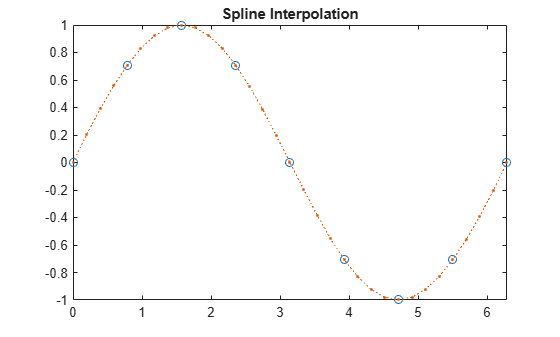Define a set of function values.

`v = [0 1.41 2 1.41 0 -1.41 -2 -1.41 0];`

Define a set of query points that fall between the default points, `1:9`. In this case, the default points are `1:9` because `v` contains `9` values.

`xq = 1.5:8.5;`

Evaluate `v` at `xq`.

`vq = interp1(v,xq);`

Plot the result.

```figure plot((1:9),v,'o',xq,vq,'*'); legend('v','vq');```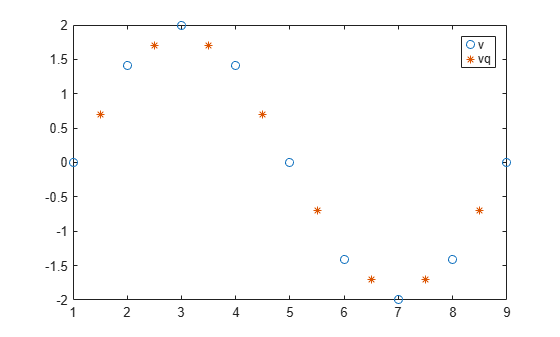Define a set of sample points.

`x = 1:10;`

Define the values of the function, $v\left(x\right)=5x+{x}^{2}i$, at the sample points.

`v = (5*x)+(x.^2*1i);`

Define the query points to be a finer sampling over the range of `x`.

`xq = 1:0.25:10;`

Interpolate `v` at the query points.

`vq = interp1(x,v,xq);`

Plot the real part of the result in red and the imaginary part in blue.

```figure plot(x,real(v),'*r',xq,real(vq),'-r'); hold on plot(x,imag(v),'*b',xq,imag(vq),'-b');```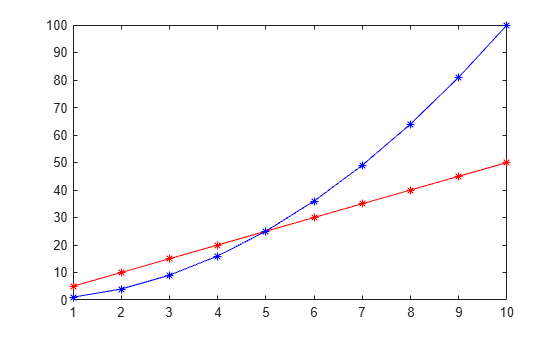Interpolate time-stamped data points.

Consider a data set containing temperature readings that are measured every four hours. Create a table with one day's worth of data and plot the data.

```x = (datetime(2016,1,1):hours(4):datetime(2016,1,2))'; x.Format = 'MMM dd, HH:mm'; T = [31 25 24 41 43 33 31]'; WeatherData = table(x,T,'VariableNames',{'Time','Temperature'})```
```WeatherData=7×2 table Time Temperature _____________ ___________ Jan 01, 00:00 31 Jan 01, 04:00 25 Jan 01, 08:00 24 Jan 01, 12:00 41 Jan 01, 16:00 43 Jan 01, 20:00 33 Jan 02, 00:00 31 ```
`plot(WeatherData.Time, WeatherData.Temperature, 'o')`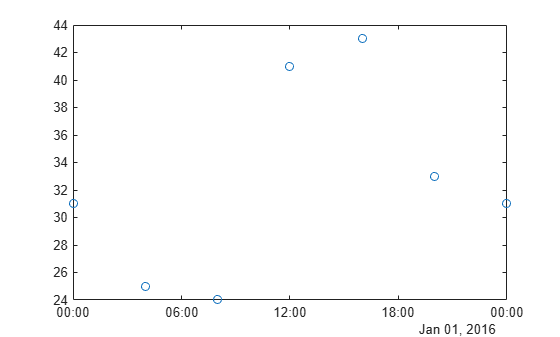Interpolate the data set to predict the temperature reading during each minute of the day. Since the data is periodic, use the `'spline'` interpolation method.

```xq = (datetime(2016,1,1):minutes(1):datetime(2016,1,2))'; V = interp1(WeatherData.Time, WeatherData.Temperature, xq, 'spline');```

Plot the interpolated points.

```hold on plot(xq,V,'r')```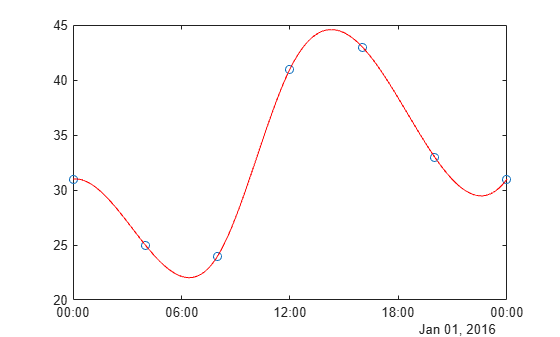Define the sample points, `x`, and corresponding sample values, `v`.

```x = [1 2 3 4 5]; v = [12 16 31 10 6];```

Specify the query points, `xq`, that extend beyond the domain of `x`.

`xq = [0 0.5 1.5 5.5 6];`

Evaluate `v` at `xq` using the `'pchip'` method.

`vq1 = interp1(x,v,xq,'pchip')`
```vq1 = 1×5 19.3684 13.6316 13.2105 7.4800 12.5600 ```

Next, evaluate `v` at `xq` using the `'linear'` method.

`vq2 = interp1(x,v,xq,'linear')`
```vq2 = 1×5 NaN NaN 14 NaN NaN ```

Now, use the `'linear'` method with the `'extrap'` option.

`vq3 = interp1(x,v,xq,'linear','extrap')`
```vq3 = 1×5 8 10 14 4 2 ```

`'pchip'` extrapolates by default, but `'linear'` does not.

Define the sample points, `x`, and corresponding sample values, `v`.

```x = [-3 -2 -1 0 1 2 3]; v = 3*x.^2;```

Specify the query points, `xq`, that extend beyond the domain of `x`.

`xq = [-4 -2.5 -0.5 0.5 2.5 4];`

Now evaluate `v` at `xq` using the `'pchip'` method and assign any values outside the domain of `x` to the value, `27`.

`vq = interp1(x,v,xq,'pchip',27)`
```vq = 1×6 27.0000 18.6562 0.9375 0.9375 18.6562 27.0000 ```

Define the sample points.

`x = (-5:5)';`

Sample three different parabolic functions at the points defined in `x`.

```v1 = x.^2; v2 = 2*x.^2 + 2; v3 = 3*x.^2 + 4;```

Create matrix `v`, whose columns are the vectors, `v1`, `v2`, and `v3`.

`v = [v1 v2 v3];`

Define a set of query points, `xq`, to be a finer sampling over the range of `x`.

`xq = -5:0.1:5;`

Evaluate all three functions at `xq` and plot the results.

```vq = interp1(x,v,xq,'pchip'); figure plot(x,v,'o',xq,vq); h = gca; h.XTick = -5:5;```The circles in the plot represent `v`, and the solid lines represent `vq`.

## Input Arguments

collapse all

Sample points, specified as a row or column vector of real numbers. The values in `x` must be distinct. The length of `x` must conform to one of the following requirements:

• If `v` is a vector, then `length(x)` must equal `length(v)`.

• If `v` is an array, then `length(x)` must equal `size(v,1)`.

Example: `[1 2 3 4 5 6 7 8 9 10]`

Example: `1:10`

Example: ```[3 7 11 15 19 23 27 31]'```

Data Types: `single` | `double` | `duration` | `datetime`

Sample values, specified as a vector, matrix, or array of real or complex numbers. If `v` is a matrix or an array, then each column contains a separate set of 1-D values.

If `v` contains complex numbers, then `interp1` interpolates the real and imaginary parts separately.

Example: `rand(1,10)`

Example: `rand(10,1)`

Example: `rand(10,3)`

Data Types: `single` | `double` | `duration` | `datetime`
Complex Number Support: Yes

Query points, specified as a scalar, vector, matrix, or array of real numbers.

Example: `5`

Example: `1:0.05:10`

Example: `(1:0.05:10)'`

Example: `[0 1 2 7.5 10]`

Data Types: `single` | `double` | `duration` | `datetime`

Interpolation method, specified as one of the options in this table.

Method

Description

Continuity

Comments

`'linear'`

Linear interpolation. The interpolated value at a query point is based on linear interpolation of the values at neighboring grid points in each respective dimension. This is the default interpolation method.

C0

• Requires at least 2 points

• Requires more memory and computation time than nearest neighbor

`'nearest'`

Nearest neighbor interpolation. The interpolated value at a query point is the value at the nearest sample grid point.

Discontinuous

• Requires at least 2 points

• Modest memory requirements

• Fastest computation time

`'next'`

Next neighbor interpolation. The interpolated value at a query point is the value at the next sample grid point.

Discontinuous

• Requires at least 2 points

• Same memory requirements and computation time as `'nearest'`

`'previous'`

Previous neighbor interpolation. The interpolated value at a query point is the value at the previous sample grid point.

Discontinuous

• Requires at least 2 points

• Same memory requirements and computation time as `'nearest'`

`'pchip'`

Shape-preserving piecewise cubic interpolation. The interpolated value at a query point is based on a shape-preserving piecewise cubic interpolation of the values at neighboring grid points.

C1

• Requires at least 4 points

• Requires more memory and computation time than `'linear'`

`'cubic'`

Cubic convolution used in MATLAB® 5.

C1

• Requires at least 3 points

• Points must be uniformly spaced

• This method falls back to `'spline'` interpolation for irregularly-spaced data

• Similar memory requirements and computation time as `'pchip'`

`'v5cubic'`

Same as `'cubic'`.

C1

`'makima'`

Modified Akima cubic Hermite interpolation. The interpolated value at a query point is based on a piecewise function of polynomials with degree at most three. The Akima formula is modified to avoid overshoots.

C1

• Requires at least 2 points

• Produces fewer undulations than `'spline'`, but does not flatten as aggressively as `'pchip'`

• Computation is more expensive than `'pchip'`, but typically less than `'spline'`

• Memory requirements are similar to those of `'spline'`

`'spline'`

Spline interpolation using not-a-knot end conditions. The interpolated value at a query point is based on a cubic interpolation of the values at neighboring grid points in each respective dimension.

C2

• Requires at least 4 points

• Requires more memory and computation time than `'pchip'`

Extrapolation strategy, specified as `'extrap'` or a real scalar value.

• Specify `'extrap'` when you want `interp1` to evaluate points outside the domain using the same method it uses for interpolation.

• Specify a scalar value when you want `interp1` to return a specific constant value for points outside the domain.

The default behavior depends on the input arguments:

• If you specify the `'pchip'`, `'spline'`, or `'makima'` interpolation methods, then the default behavior is `'extrap'`.

• All other interpolation methods return `NaN` by default for query points outside the domain.

Example: `'extrap'`

Example: `5`

Data Types: `char` | `string` | `single` | `double`

## Output Arguments

collapse all

Interpolated values, returned as a scalar, vector, matrix, or array. The size of `vq` depends on the shape of `v` and `xq`.

Shape of vShape of xqSize of VqExample
VectorVector`size(xq)`If `size(v) = [1 100]`
and ```size(xq) = [1 500]```,
then `size(vq) = [1 500]`.
VectorMatrix
or N-D Array
`size(xq)`If `size(v) = [1 100]`
and ```size(xq) = [50 30]```,
then ```size(vq) = [50 30]```.
Matrix
or N-D Array
Vector`[length(xq) size(v,2),...,size(v,n)]`If `size(v) = [100 3]`
and ```size(xq) = [1 500]```,
then ```size(vq) = [500 3]```.
Matrix
or N-D Array
Matrix
or N-D Array
`[size(xq,1),...,size(xq,n),... size(v,2),...,size(v,m)]`If `size(v) = [4 5 6]`
and ```size(xq) = [2 3 7]```,
then ```size(vq) = [2 3 7 5 6]```.

Piecewise polynomial, returned as a structure that you can pass to the `ppval` function for evaluation.

## More About

collapse all

### Akima and Spline Interpolation

The Akima algorithm for one-dimensional interpolation, described in  and , performs cubic interpolation to produce piecewise polynomials with continuous first-order derivatives (C1). The algorithm preserves the slope and avoids undulations in flat regions. A flat region occurs whenever there are three or more consecutive collinear points, which the algorithm connects with a straight line. To ensure that the region between two data points is flat, insert an additional data point between those two points.

When two flat regions with different slopes meet, the modification made to the original Akima algorithm gives more weight to the side where the slope is closer to zero. This modification gives priority to the side that is closer to horizontal, which is more intuitive and avoids the overshoot. (The original Akima algorithm gives equal weights to the points on both sides, thus evenly dividing the undulation.)

The spline algorithm, on the other hand, performs cubic interpolation to produce piecewise polynomials with continuous second-order derivatives (C2). The result is comparable to a regular polynomial interpolation, but is less susceptible to heavy oscillation between data points for high degrees. Still, this method can be susceptible to overshoots and oscillations between data points.

Compared to the spline algorithm, the Akima algorithm produces fewer undulations and is better suited to deal with quick changes between flat regions. This difference is illustrated below using test data that connects multiple flat regions.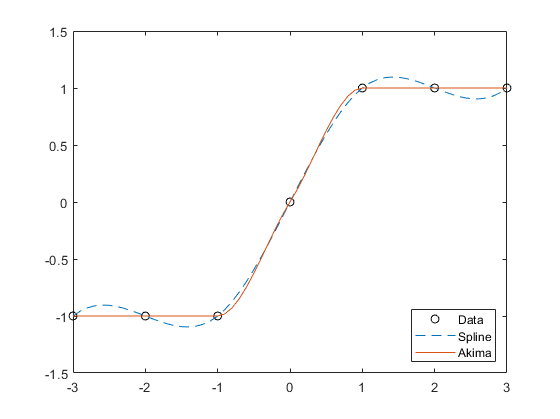## Compatibility Considerations

expand all

Behavior changed in R2020b

 Akima, Hiroshi. "A new method of interpolation and smooth curve fitting based on local procedures." Journal of the ACM (JACM) , 17.4, 1970, pp. 589-602.

 Akima, Hiroshi. "A method of bivariate interpolation and smooth surface fitting based on local procedures." Communications of the ACM , 17.1, 1974, pp. 18-20.

Download ebook